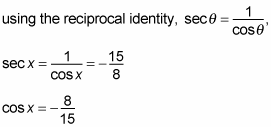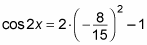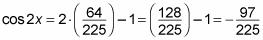##### Trigonometry Workbook For DummiesYou can use three different formulas to find the value for cos 2x
, the cosine of a double-angle. As a result, your job is to choose which one best fits into the problem. The double-angle formula for cosine comes from the sum formula, just like the double-angle formula for sine. If you can't remember the double-angle formula but you can remember the sum formula, just rewrite cos(2x) as cos(x + x).

Because using the sum formula for cosine yields cos 2x = cos2 x – sin2 x, you have two additional ways to express this by using Pythagorean identities:

• You can replace sin2 x with (1 – cos2 x) and simplify to get cos 2x = 2 cos2 x – 1.

• You can replace cos2 x with (1 – sin2 x) and simplify to get cos 2x = 1 – 2 sin2 x.

Following are the possible formulas for the double-angle of cosine:
• cos 2x = cos2 x – sin2 x

• cos 2x = 2 cos2 x – 1

• cos 2x = 1 – 2 sin2 x

Looking at what you're given and what you're asked to find usually will lead you toward the right formula. And hey, if you don't pick the right one at first, you have two more to try!

Here's an example problem: If sec x = –15/8, find the exact value of cos 2x if x is in quadrant II of the coordinate plane. Follow these steps to solve:

1. Use the reciprocal identity to change secant to cosine.

Because secant doesn't appear in any of the possible formula choices, you have to complete this step first. Therefore,2. Choose the appropriate double-angle formula.

Because you now know the cosine value, you should choose the second double-angle formula for this problem:

cos 2x = 2 cos2 x – 1

3. Substitute the information you know into the formula.

You can plug cosine into the equation:4. Simplify the formula to solve.